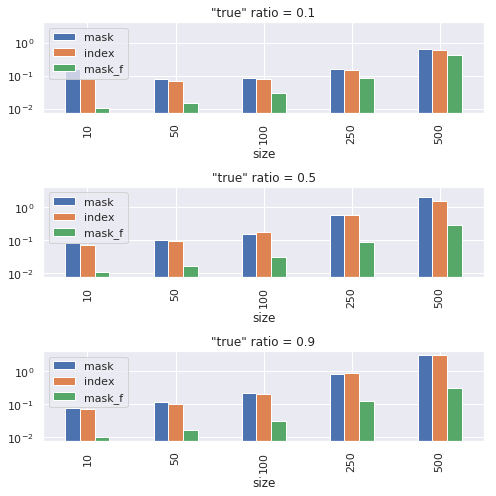# Operations on subset of tensor, which to use?

I often need to conduct scalar operation (e.g. add or multiply) on a subset of tensor elements, where the subset is specified by another tensor in a form of index or mask.
There are multiple ways of doing this, and I would like to know which to use or which is better.
I myself conducted a few experiments of speed comparison (details below).
In short, the result was that indexing (using long or byte) tends to be slower than updating the whole tensor.

Question

I would like to know pros and cons of different ways to update a subset of tensor. In particular, should we avoid subset updating in a form `x[cond] = ...` if other options are available?

Below describes the experiments that I made.

Operation on subset of rows

Let `X` be a float tensor on which changes are made, and a is a scalar. Suppose we want to add a to as subset rows of `X`.
As far as I know, there are following ways of doing this:

• `X[idx] += a`, where `idx` is a long 1-D tensor of indices.
• `X[mask] += a`, where `mask` is a byte 1-D tensor of conditions.
• `X += mask_f * a`, where `mask_f` is a float 1-D tensor of conditions.

Graph below is the time for 1000 operations. “true” ratio is the fraction of rows satisfying the condition. Size is the size of rows and columns of `X`.
`X += mask_f * a` tends to be faster for many cases, but “indexing” may outperform with small `p` (only few rows satisfy condition) and large tensor size.Operation on subset of elements

Now suppose we have a element-wise condition in a form of mask. And we would like to add `a` only to the elements of `X` where condition is satisfied. I know the following two ways.

• `X[mask] += a`, where `mask` is a byte tensor of conditions with same size as `X`.
• `X += mask_f * a`, where `mask_f` is a float tensor of conditions with same size as `X`.

Again, `X += mask_f * a` is faster.Code to reproduce (up to randomness).

``````import timeit
import random
import torch
import numpy as np
number = 1000
y = 4.0
out = []

for p in [0.1, 0.5, 0.9]:
for s in [10, 50, 100, 250, 500]:
x0 = np.random.random((s, s))
mask = np.array(random.choices([True, False], weights=[p, 1-p], k=s), dtype=np.uint8)
idx = torch.tensor(idx, dtype=torch.long)

x = torch.tensor(x0, dtype=torch.float32)
t1 = timeit.timeit("x[mask] += y", setup=setup, number=number)
x1 = x.numpy()

x = torch.tensor(x0, dtype=torch.float32)
t2 = timeit.timeit("x[idx] += y", setup=setup, number=number)
x2 = x.numpy()

x = torch.tensor(x0, dtype=torch.float32)
t3 = timeit.timeit("x += mask_f * y", setup=setup, number=number)
x3 = x.numpy()
assert np.all(x1 == x2)
assert np.all(x1 == x3)
out.append([s, p, t1, t2, t3])

import pandas as pd
import matplotlib.pyplot as plt
import seaborn as sns
sns.set()

df = pd.DataFrame(out, columns=["size", "true_ratio",
fig, axes = plt.subplots(3, 1, figsize=(7, 7), sharey=True)
for i, p in enumerate(df.index.levels):
ax = axes[i]
df.loc[p].plot(kind="bar", ax=ax)
ax.set_yscale("log")
ax.set_title('"true" ratio = ' + str(p))
fig.tight_layout()

y = 4.0
number = 1000
out = []
for p in [0.1, 0.5, 0.9]:
for s in [10, 50, 100, 250, 500]:
x0 = np.random.random((s, s))
mask = np.array(random.choices([True, False], weights=[p, 1-p], k=s*s), dtype=np.uint8).reshape((s, s))

x = torch.tensor(x0, dtype=torch.float32)
t1 = timeit.timeit("x[mask] += y", setup=setup, number=number)
x1 = x.numpy()

x = torch.tensor(x0, dtype=torch.float32)
t2 = timeit.timeit("x += mask_f * y", setup=setup, number=number)
x2 = x.numpy()

assert np.all(x1 == x2)
out.append([s, p, t1, t2])